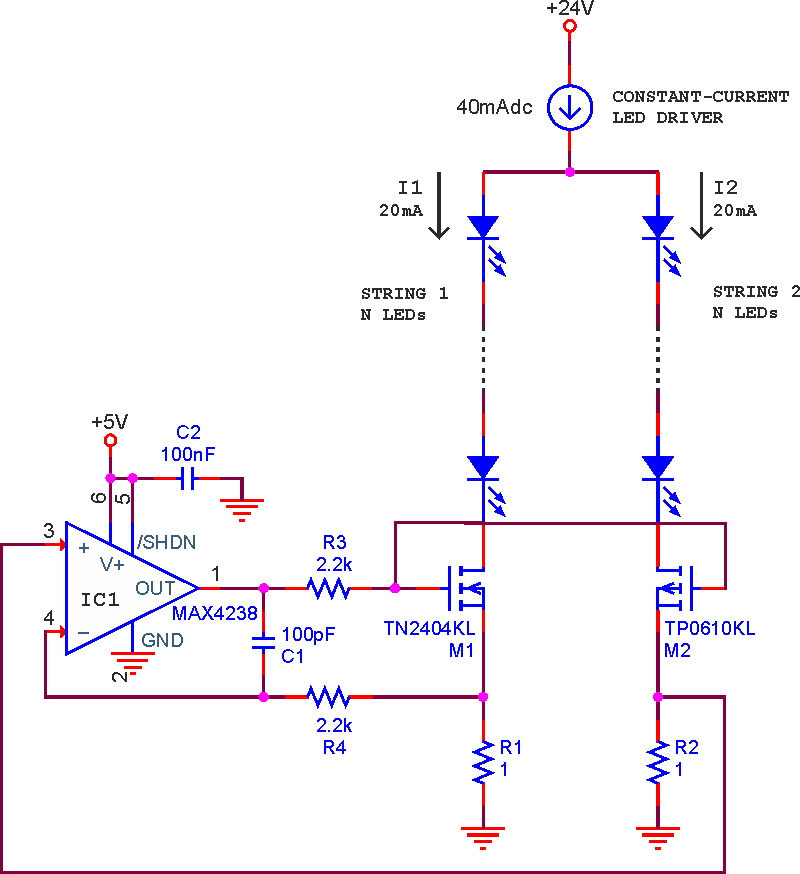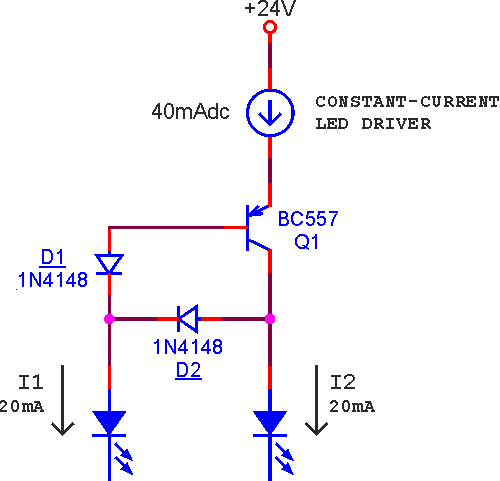LED strings driven by current source/mirror

Maxim MAX4238

Luca Bruno

EDN

The Design Idea in Figure 1 is a precision current mirror used to drive two strings of five white LEDs.Figure 1. Current source/mirror driving two LED strings.

The op-amp has "double" negative feedback (since M2 inverts the signal at the non-inverting input). The voltage drops across R1 and R2 are equal, halving the LED driver current. MOSFET M2 works with a constant source-drain voltage (equal to the sum of VGS1 + VGS2) while M1 absorbs the mismatch of the voltage drops of the two strings, even in the case where an LED is shorted.

To protect the LED string from overcurrent due to an open in one of the strings, you can add two diodes and one transistor as shown in Figure 2 and described in my previous Design Idea (Ref. 1).Figure 2. Protection against opens.

Capacitor C1 along with resistors R3 and R4 ensure stability of the op-amp. The voltage drop across R1 and R2 must be suitably greater than the maximum offset voltage of the op-amp to achieve good accuracy in dividing the LED driver current. The current ratio I1:I2 is equal only to the resistor ratio R1:R2.

The circuit is also suitable for driving power LEDs. In this case you should use MOSFETs with low threshold voltage to limit power dissipation, or you could replace them with a complementary pair of BJTs.

EDN